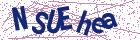with the collaboration of Iranian Society of Mechanical Engineers (ISME)

Document Type : Research Article

Authors

1 Department of Biosystems Engineering Shiraz University

2 Department of Gas Engineeirng Shiraz University

Abstract

Introduction
Drying shrimp is one of the storage methods that, while increasing the shelf life, leads to the production of a diverse product with different uses, from consumption as snacks to use as one of the main components of foods. Drying is preferred over other preservation methods due to advantages such as long shelf life, microbial stability, easy consumption, reduced transportation costs, high added value, and product diversity.
In order to simulate all these processes and thus obtain information such as their shelf life as well as the energy required for the processes, it is necessary to determine the initial and final temperature of the product, its geometry and dimensions, as well as their thermo-physical characteristics. Simulation of different drying processes requires accurate estimation of effective moisture diffusion coefficient. This parameter is highly dependent on temperature and humidity, which level of dependence is usually shown by an equation with an Arrhenius structure as an empirical function of humidity and temperature, or by considering the activation energy.
It is necessary to have sufficient knowledge about heat and mass transfer characteristics, such as diffusion or penetration coefficient and heat transfer coefficient to estimate the final temperature and drying time. In this study, the drying process of peeled farmed shrimp (Litopenaeus vannamei) in a convective hot air dryer, and variations in related parameters such as shrinkage and effective moisture diffusion coefficient were studied.
Materials and Methods
A drying device was built to conduct experimental experiments on drying shrimp samples. Experiments were performed on samples of shrimp meat slices at temperatures of 40, 50 and 60 degrees Celsius and constant air velocity of 1.5 m/s. The basis of experimental drying models was on diffusion theory. In these models, it was assumed that the resistance to the diffusion of moisture occurs from the outer layer of food. In most cases, Fick's second law is used to describe the phenomenon of moisture penetration.
In the present study, the standard method of measuring volume changes by immersion method (in toluene) was used to determine the sample volume changes. During drying time, the volume of the samples were measured at intervals of 45 minutes, and their volume changes were calculated. To measure the moisture content of the samples, at the beginning of each test, the weight of the samples were recorded with a digital scale with an accuracy of ±0.001 g. Then, during drying time of the samples, simultaneously with the volume measurement, the samples were weighed.
The most common way to model shrinkage during the drying process is to obtain an empirical relationship between shrinkage and moisture, for which linear and non-linear models were used. In most cases, effective permeability is defined as a function of humidity and temperature. For this purpose, by using curve fitting methods on the data obtained from experimental tests and including the Arrhenius equation (for most food items), the appropriate function was extracted.
Results and discussion
With considering the results of statistical indices, the linear model was the best model for expressing shrinkage changes versus moisture ratio changes among the experimental models considered for shrinkage and drying kinetics, and Weibull distribution was the best model for expressing moisture ratio-time variations. A moisture dependent experimental model was used to express the changes in the apparent density of shrimp, by which the range of 1017-1117 kg/m3 was computed. Moreover, to express the effect of moisture content and temperature on the effective diffusion coefficient of shrimp, an Arrhenius equation was derived. According to the results, the variations in the effective diffusion coefficient of shrimp ranged between 0.08 ×10-9 m2/s to 7.39×10-9 m2/s. While deriving the effective diffusion coefficient, the influence of the number Fick's second law terms on moisture ratio variation was studied, and it was found that number of terms more than 100 could not change the model outputs, considerably.
Conclusion
Among the evaluated shrinkage models, a linear model with the highest coefficient of determination (R2) and the lowest root mean square error and sum of square error (SSE) was the best model for interpreting shrinkage at the tested temperature levels. The experimental model of Weibull distribution in the evaluated temperature range, was the most suitable model to express the changes in the moisture ratio of shrimp meat slices against time. Arrhenius model for predicting the effective diffusion coefficient of shrimp slices predicted the changes in the diffusion coefficient of shrimp meat slices with respect to temperature and moisture content in the evaluated temperature range.

Keywords

Main Subjects